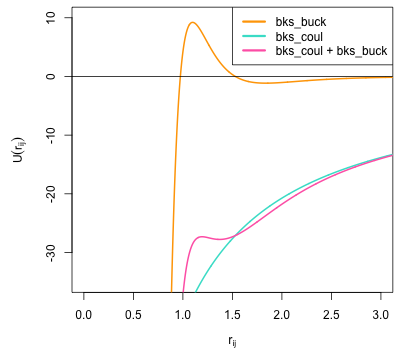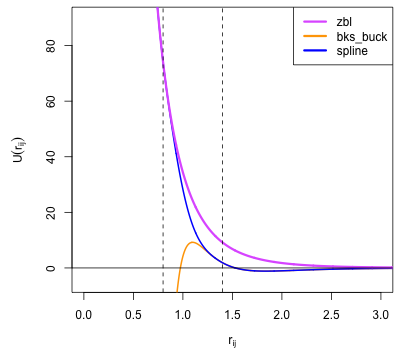# Pair Potential Tabulation¶

Pair potentials are tabulated using the convenience function atsim.potentials.writePotentials(). This function is supplied with a list of Potential objects, which have PotentialInterface.energy() and PotentialInterface.force() methods called during tabulation to obtain potential-energy as a function of separation and its derivative respectively.

## atsim.potentials.writePotentials()¶

The atsim.potentials.writePotentials() function is used to tabulate pair-potentials for the supported simulation codes.

The process by which writePotentials() is used can be summmarised as follows:

1. Define python functions describing energy of interactions (see Example: Instantiating atsim.potentials.Potential Objects and Predefined Potential Forms)
2. Wrap these functions in Potential objects.
3. Call writePotentials() with list of Potential objects choosing:
• Tabulation type
• Potential cut-off
• Number of rows in tabulation
• Python file like object into which data should be written.

## Potential Objects¶

Potential objects should implement the following interface:

class PotentialInterface
speciesA

(str): Attribute giving first species in pair being described by pair-potential

speciesB

(str): Attribute giving second species in pair described by pair-potential

energy(self, r)

Calculate energy between atoms for given separation.

Parameters: r (float) – Separation between atoms of speciesA and speciesB Energy in eV for given separation. float
force(self, r)

Calculate force (-dU/dr) for interaction at a given separation.

Parameters: r (float) – Separation -dU/dr at r in eV per Angstrom. float

In most cases the atsim.potentials.Potential class provided in atsim.potentials can be used. This wraps a python callable that returns potential energy as a function of separation to provide the values returned by the atsim.potentials.Potential.energy() method. The forces calculated by the atsim.potentials.Potential.force() method are obtained by taking the numerical derivative of the wrapped function.

### Example: Instantiating atsim.potentials.Potential Objects¶

The following example shows how a Born-Mayer potential function can be described and used to create a Potential object for the interaction between Gd and O. The Born-Mayer potential is given by:

$U_{\text{Gd-O}}(r_{ij}) = A \exp\left( \frac{- r_{ij}}{{\rho}} \right)$

Where $$U_{\text{Gd-O}}(r_{ij})$$ is the potential energy between atoms $$i$$ and $$j$$ of types Gd and O, separated by $$r_{ij}$$. The parameters $$A$$ and $$\rho$$ will be taken as 1000.0 and 0.212.

The Gd-O potential function can be defined as:

import math
from atsim.potentials import Potential

def bornMayer_Gd_O(rij):
energy = 1000.0 * math.exp(-rij/0.212)
return energy


This is then passed to Potential‘s constructor along with the species names:

pot = Potential('Gd', 'O', bornMayer_Gd_O)


The energy and force at a separation of 1Å can then be obtained by calling the energy() and force() methods:

>>> pot.energy(1.0)
8.942132960434881
>>> pot.force(1.0)
42.17987245936639


## Predefined Potential Forms¶

In the previous example (instantiate_potential_object), a function named bornMayer_Gd_O() was defined for a single pair-interaction, with the potential parameters hard-coded within the function. Explicitly defining a function for each interaction quickly becomes tedious for anything but the smallest parameter sets. In order to make the creation of functions using standard potential forms easier, a set of function factories are provided within the atsim.potentials.potentialsforms module.

Using the potentialsforms module, the function:

import math

def bornMayer_Gd_O(rij):
energy = 1000.0 * math.exp(-rij/0.212)
return energy


can be rewritten as:

from atsim.potentials import potentialforms
bornMayer_Gd_O = potentialsforms.bornmayer(1000.0, 0.212)


See API reference for list of available potential forms: atsim.potentials.potentialforms

### Combining Potential Forms¶

Pair interactions are often described using a combination of standard potential forms. This was seen for the Basak potentials used within the Quick-Start example, where the oxygen-uranium pair potential was the combination of a Buckingham and Morse potential forms.

Such potential combinations can be made using the plus() function from the atsim.potentials module:

## Spline Interpolation¶

The SplinePotential class can be used to smoothly interpolate between two different potential forms within the same potential curve: one potential function acts below a given cutoff (referred to as the detachment point) and the other potential function takes over at larger separations (acting above a second cutoff called the attachment point). An exponential interpolating spline acts between the detachment and attachment points to provide a smooth transition between the two potential curves.

The SplinePotential class aims to automatically determine spline coefficients such that the resultant, interpolated, potential curve is continuous in its first and second derivatives. The analytical form of the interpolating spline is (where $$r_{ij}$$ is interatomic separation and $$B_{0..5}$$ are the spline coefficients calculated by the SplinePotential class):

$U(r_{ij}) = \exp \left( B_0 + B_1 r_{ij} + B_2 r_{ij}^2 + B_3 r_{ij}^3 + B_4 r_{ij}^4 + B_5 r_{ij}^5 \right)$

The SplinePotential has a number of applications, for example:

• certain potential forms can become attractive in an unphysical manner at small separations (an example is the so-called Buckingham catastrophe); SplinePotential can be used to combine an appropriate repulsive potential at short separations whilst still using the other form for equilibrium and larger separations.
• similarly different potential forms may be better able to express certain separations than others. For instance the zbl() potential is often used to describe the high energy interactions found in radiation damage cascades but must be combined with another potential to describe equilibrium properties.

### Example: Splining ZBL Potential onto Buckingham Potential¶

As mentioned above, for certain parameterisations, popular potential forms can exhibit unphysical behaviour for some interatomic separations. A popular model for the description of silicate and phosphate systems is that due to van Beest, Kramer and van Santen (the BKS potential set) . In the current example, the Si-O interaction from this model will be considered. This uses the Buckingham potential form with the following parameters:

• A = 18003.7572 eV
• $$\rho$$ = 0.205204 Å
• C = 133.5381 eV $$Å^6$$
• Charges:
• Si = 2.4 e
• O = -1.2 e

The following plot shows the combined coulomb and short-range contributions for this interaction plotted as a function of separation. The large C term necessary to describe the equilibrium properties of silicates means that as $$r_{ij}$$ gets smaller, the $$\frac{C}{r_{ij}^6}$$ overwhelms the repulsive Born-Mayer component of the Buckingham potential meaning that it turns over. This creates only relatively shallow minimum arround the equilibrium Si-O separation. Within simulations containing high velocities (e.g. high temperatures or collision cascades) atoms could easily enter the very negative, attractive portion of the potential at low $$r_{ij}$$ - effectively allowing atoms to collapse onto each other. In order to overcome this deficiency a ZBL potential will be splined onto the Si-O interaction within this example.Plot of BKS Si-O potential showing the short-range (bks_buck) component, electrostatic (bks_coul) and the effective Si-O interaction (bks_buck + bks_coul). This shows that this potential turns over at small separations making it unsuitable for use where high energies may be experienced such as high-temperature or radiation damage cascade simulations.

The first step to using SplinePotential is to choose appropriate detachment and attachment points. This is perhaps best done plotting the two potential functions to be splined. The potentials module contains the convenience functions atsim.potentials.plot() and atsim.potentials.plotToFile() to make this task easier. The following piece of code first defines the ZBL and Buckingham potentials before plotting them into the files zbl.dat and bks_buck.dat. These files each contain two, space delimited, columns giving $$r_{ij}$$ and energy, and may be easily plotted in Excel or GNU Plot.

from atsim.potentials import potentialforms
import atsim.potentials

zbl = potentialforms.zbl(14, 8)
bks_buck = potentialforms.buck(18003.7572, 1.0/4.87318, 133.5381)

atsim.potentials.plot( 'bks_buck.dat', 0.1, 10.0, bks_buck, 5000)
atsim.potentials.plot( 'zbl.dat', 0.1, 10.0, zbl, 5000)


Plotting these files show that detachmentX and attachmentX values of 0.8 and 1.4 may be appropriate. The zbl and bks_buck functions can then be splined between these points as follows:

spline = atsim.potentials.SplinePotential(zbl, bks_buck, 0.8, 1.4)


Plot data can then be created for the combined functions with the interpolating spline:

atsim.potentials.plot( 'spline.dat', 0.1, 10.0, spline, 5000)


Plotting the splined Si-O potential together with the original buck and zbl functions allows the smooth transition between the two functions to be observed, as shown in the following function:Plot of BKS Si-O interaction showing the short-range (buck) and ZBL functions plotted with the curve generated by SplinePotential (spline). This joins them with a an interpolating spline acting between the detachment point at $$r_{ij} = 0.8$$ and re-attachment point at $$r_{ij} = 1.4$$ shown by dashed lines.

Finally, the potential can be tabulated in a format suitable for LAMMPS using atsim.potentials.writePotentials() :

bks_SiO = atsim.potentials.Potential('Si', 'O', spline)
with open('bks_SiO.lmptab', 'wb') as outfile:
atsim.potentials.writePotentials('LAMMPS', [bks_SiO], 10.0, 5000, out = outfile)

  Van Beest, B. W. H., Kramer, G. J., & van Santen, R. A. (1990). Force fields for silicas and aluminophosphates based on ab initio calculations. Physical Review Letters , 64 (16), 1955–1958. http://dx.doi.org/doi:10.1103/PhysRevLett.64.1955